# R S Aggarwal Solutions for Class 10 Maths Chapter 6 Coordinate Geometry Exercise 6B

R S Aggarwal Solutions for Class 10 Maths Chapter 6 Coordinate Geometry helps students get mastering on the listed concepts. Chapter 6 exercise 6B is all about section formula, midpoint formula and centroid of a triangle. With the help of various solved problems students can clear their doubts and able to solve any of the problems at their own pace. Download Class 10 Maths Chapter 6 R S Aggarwal Solutions and start practice offline to score good marks in your exams.

## Download PDF of R S Aggarwal Solutions for Class 10 Maths Chapter 6 Coordinate Geometry Exercise 6B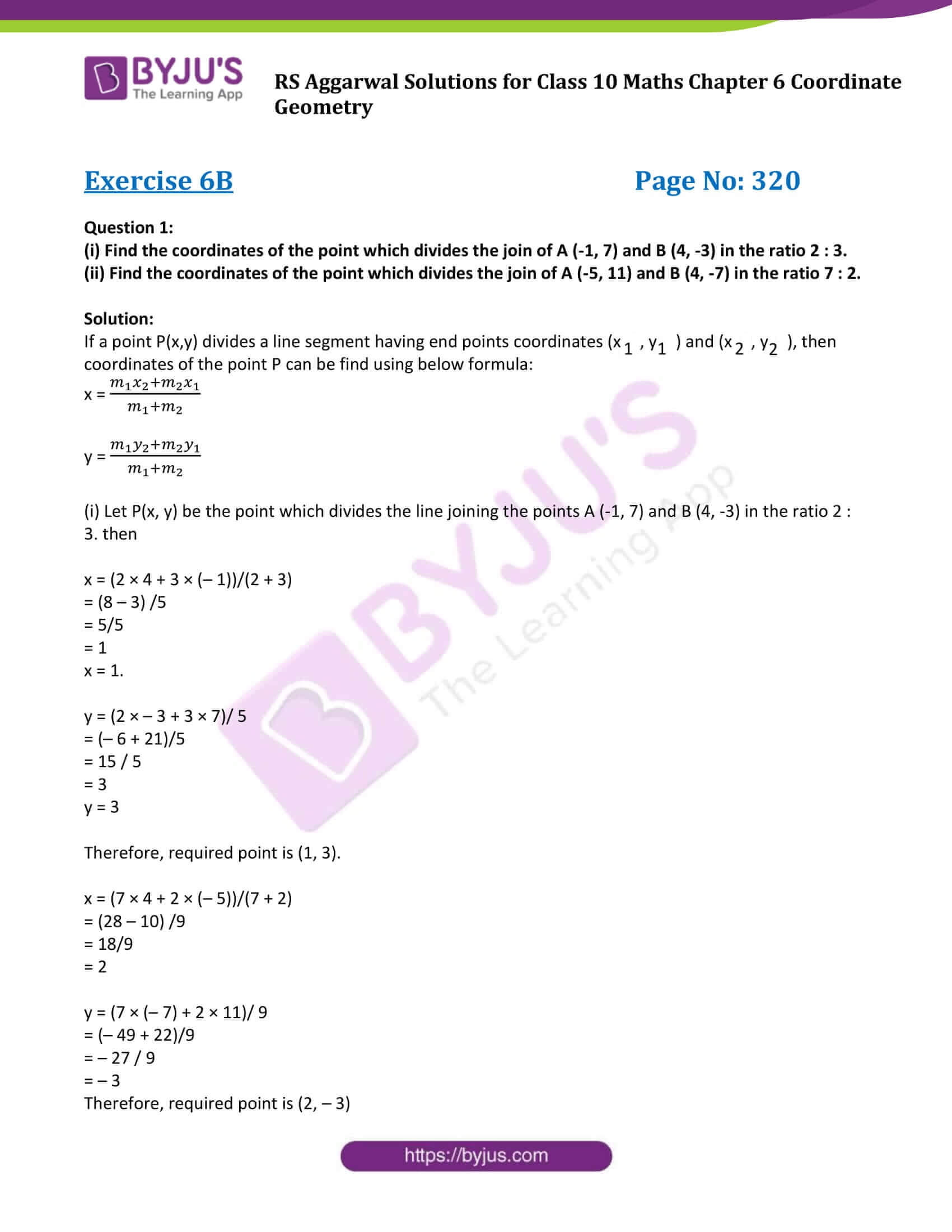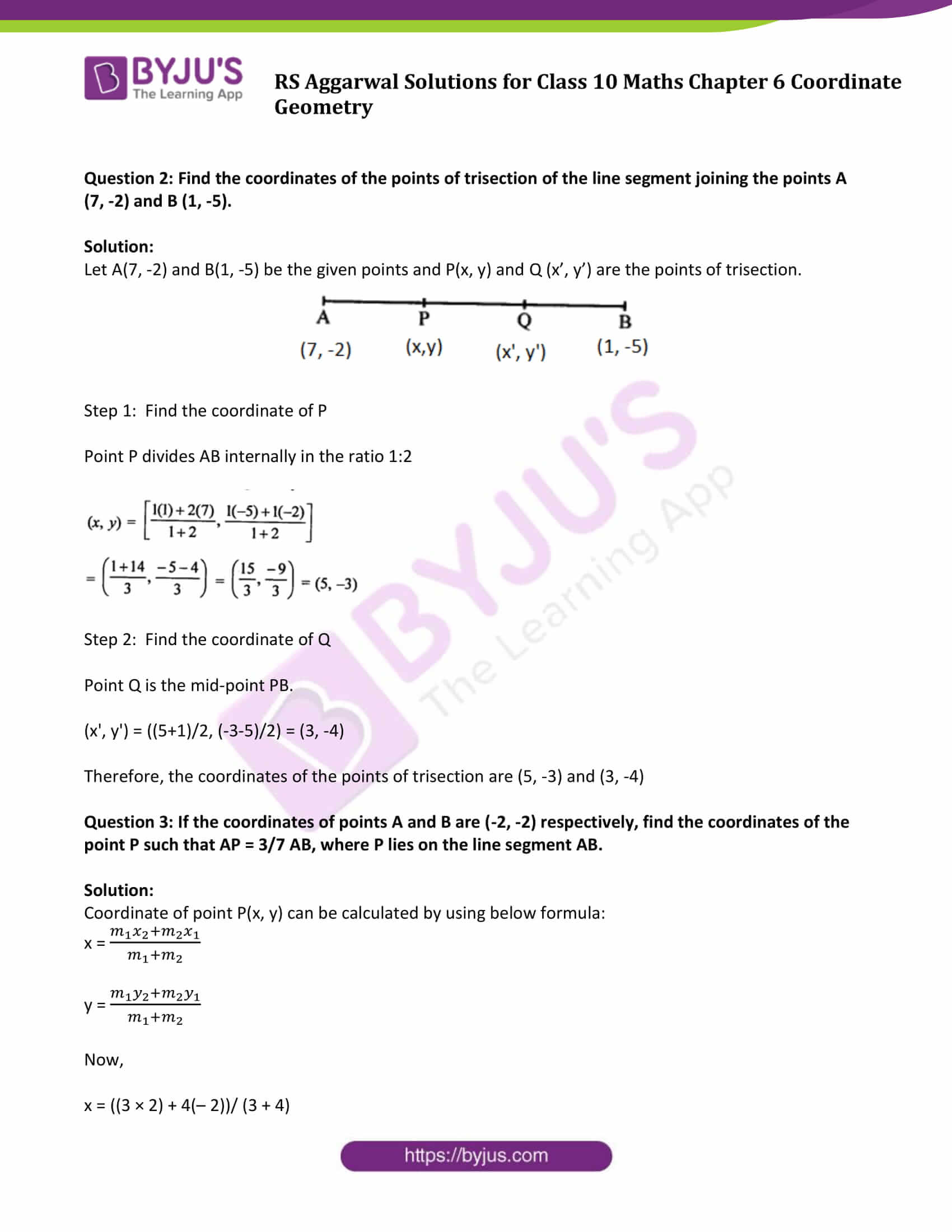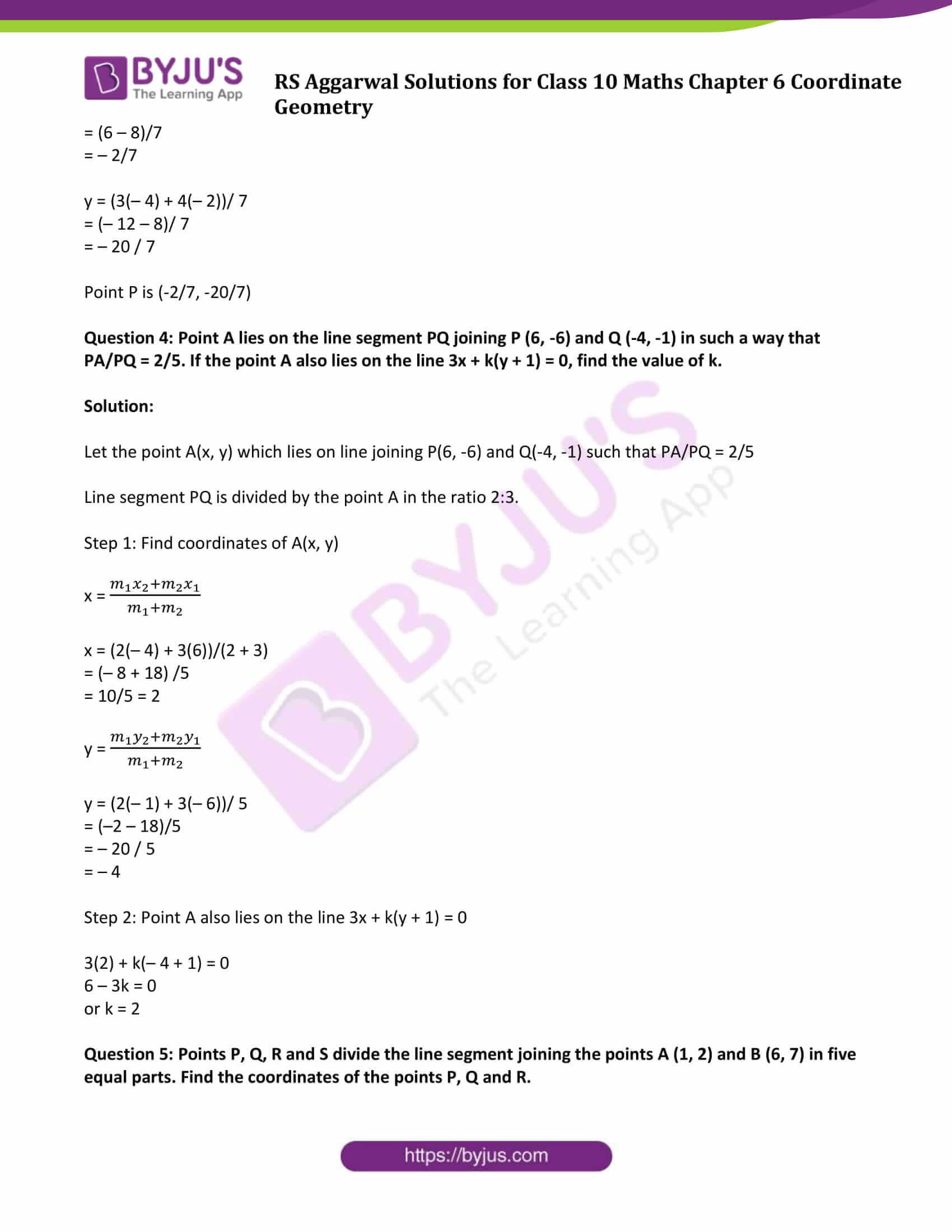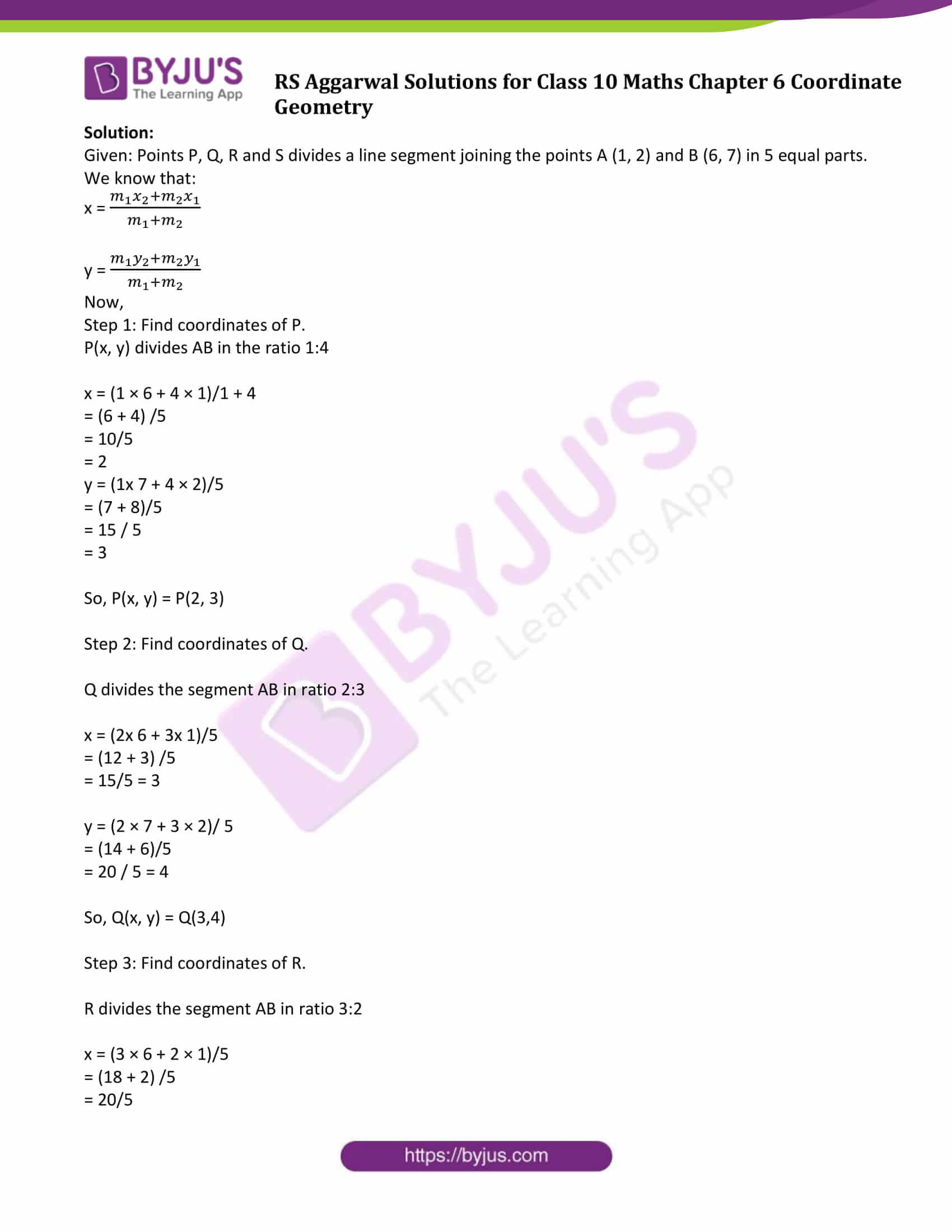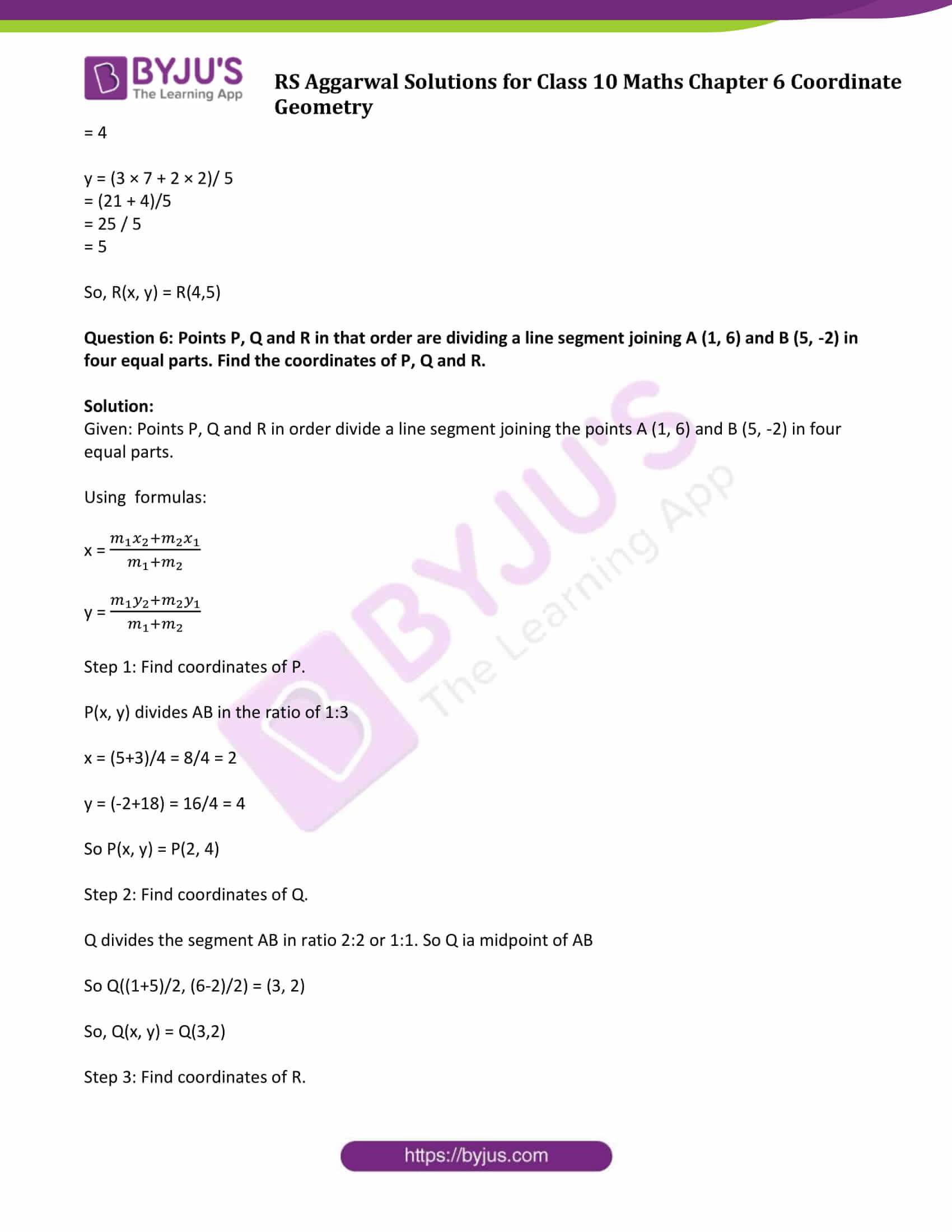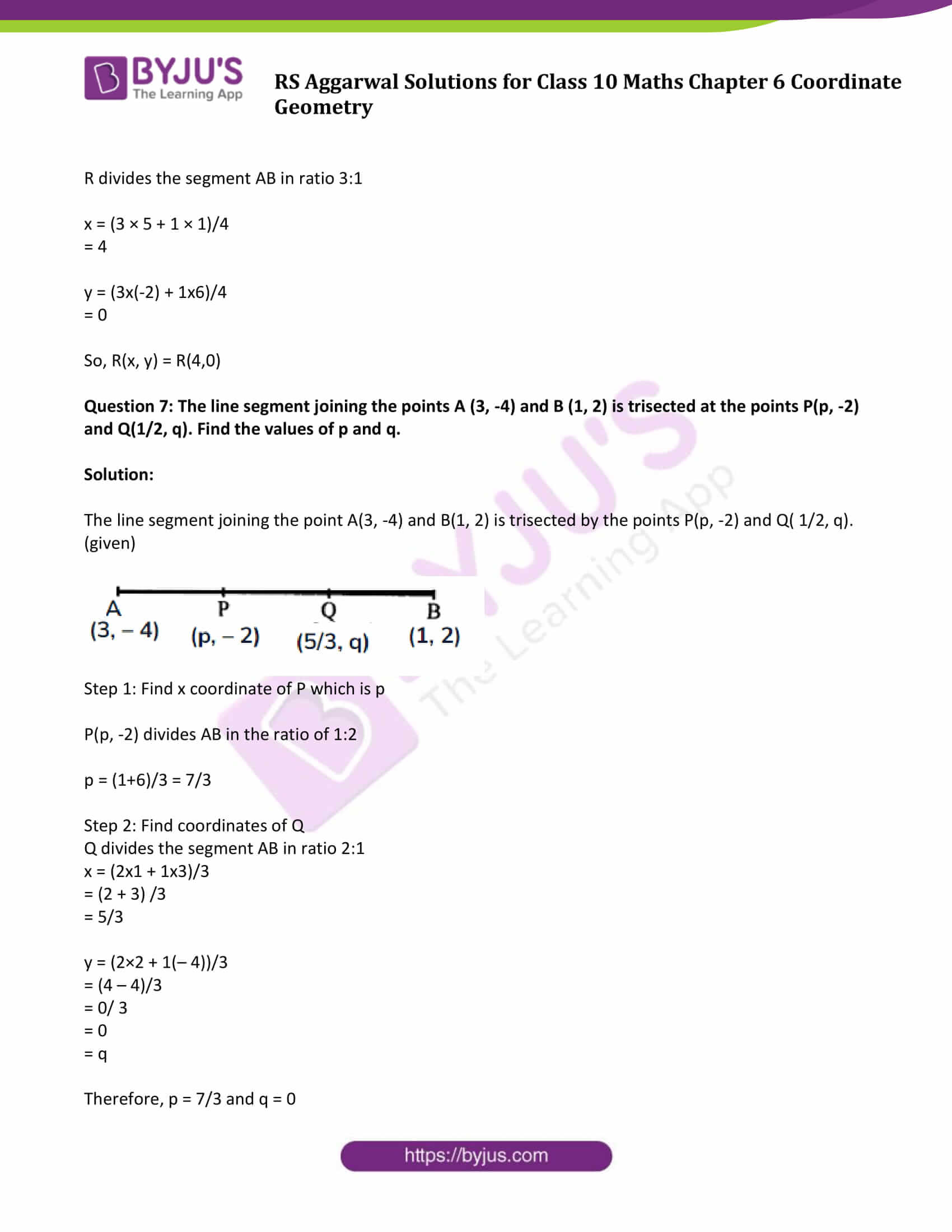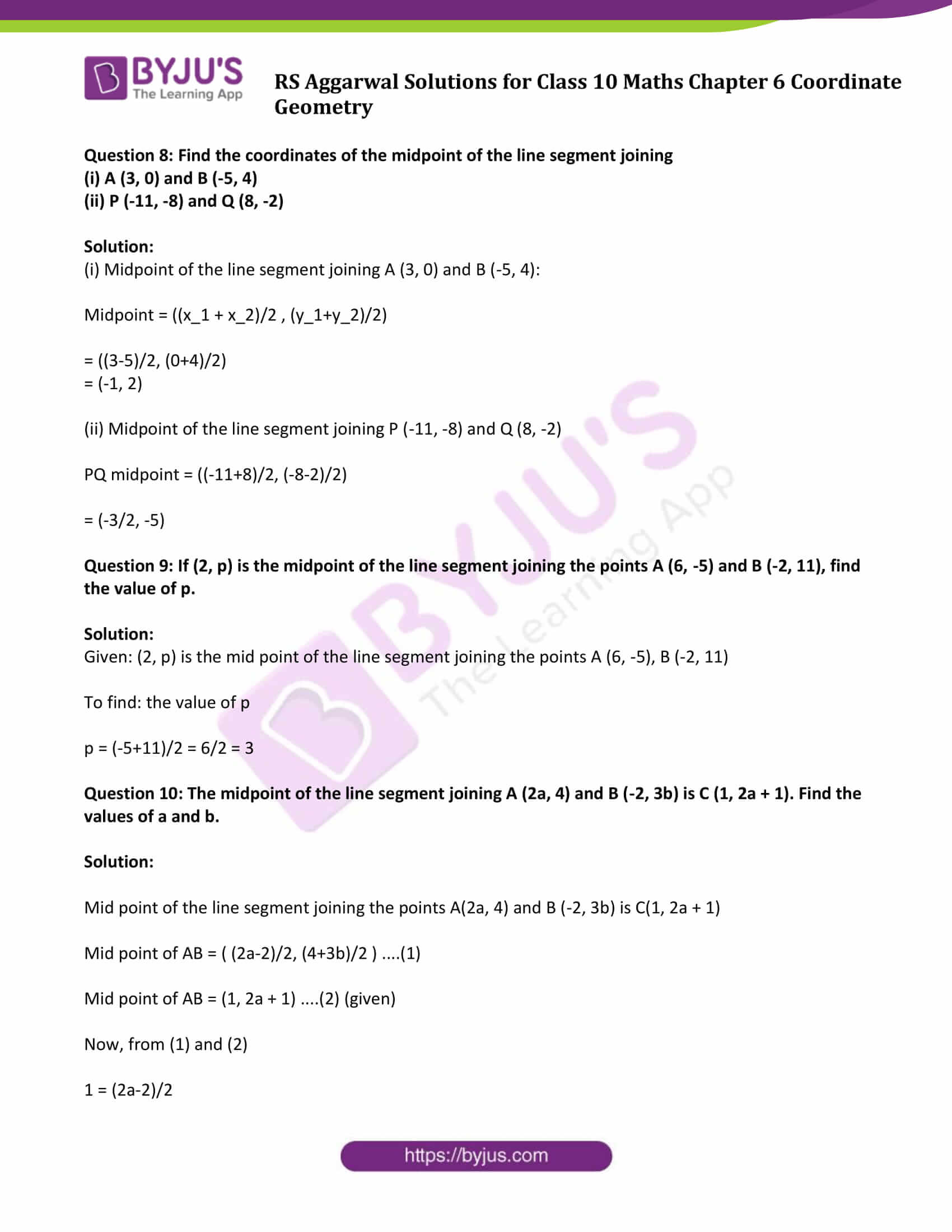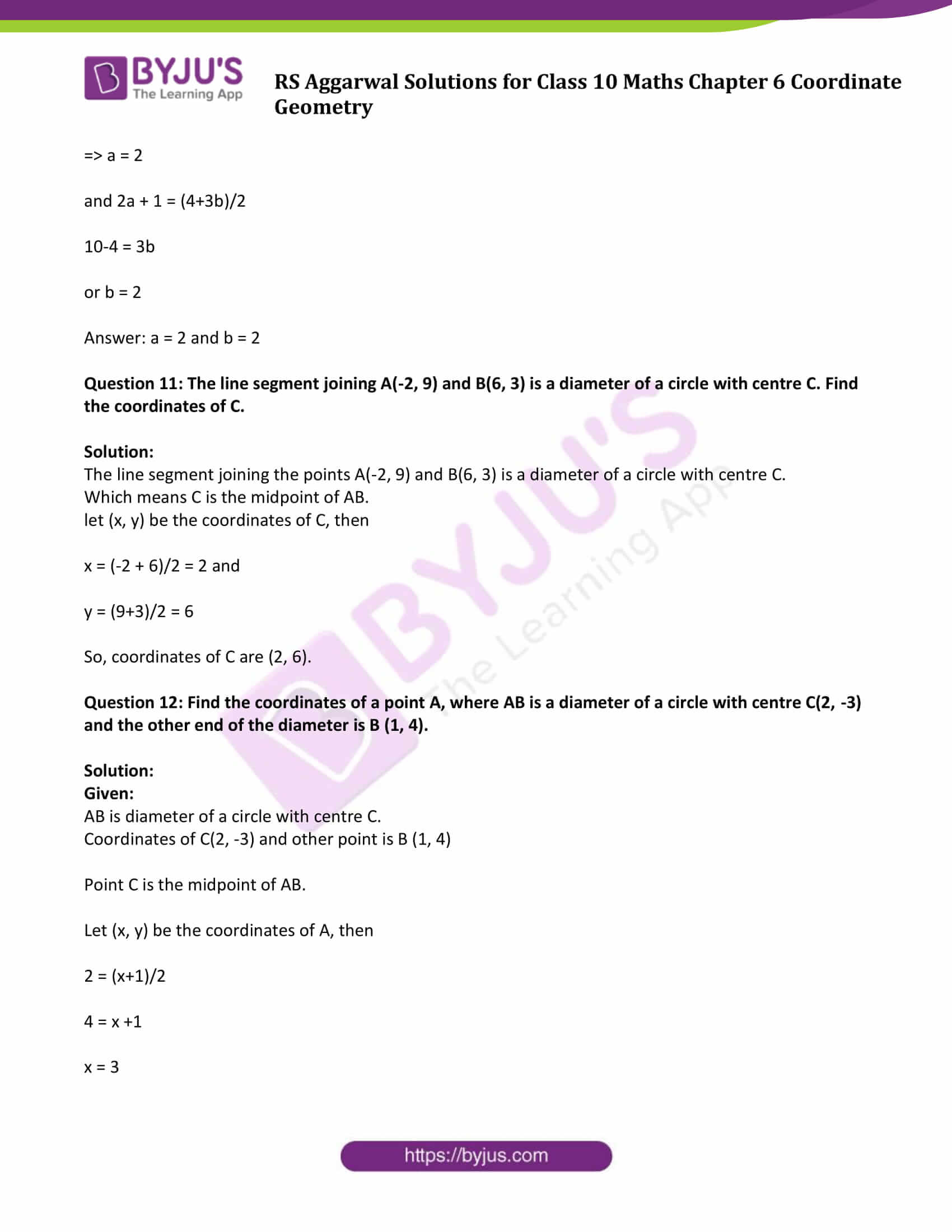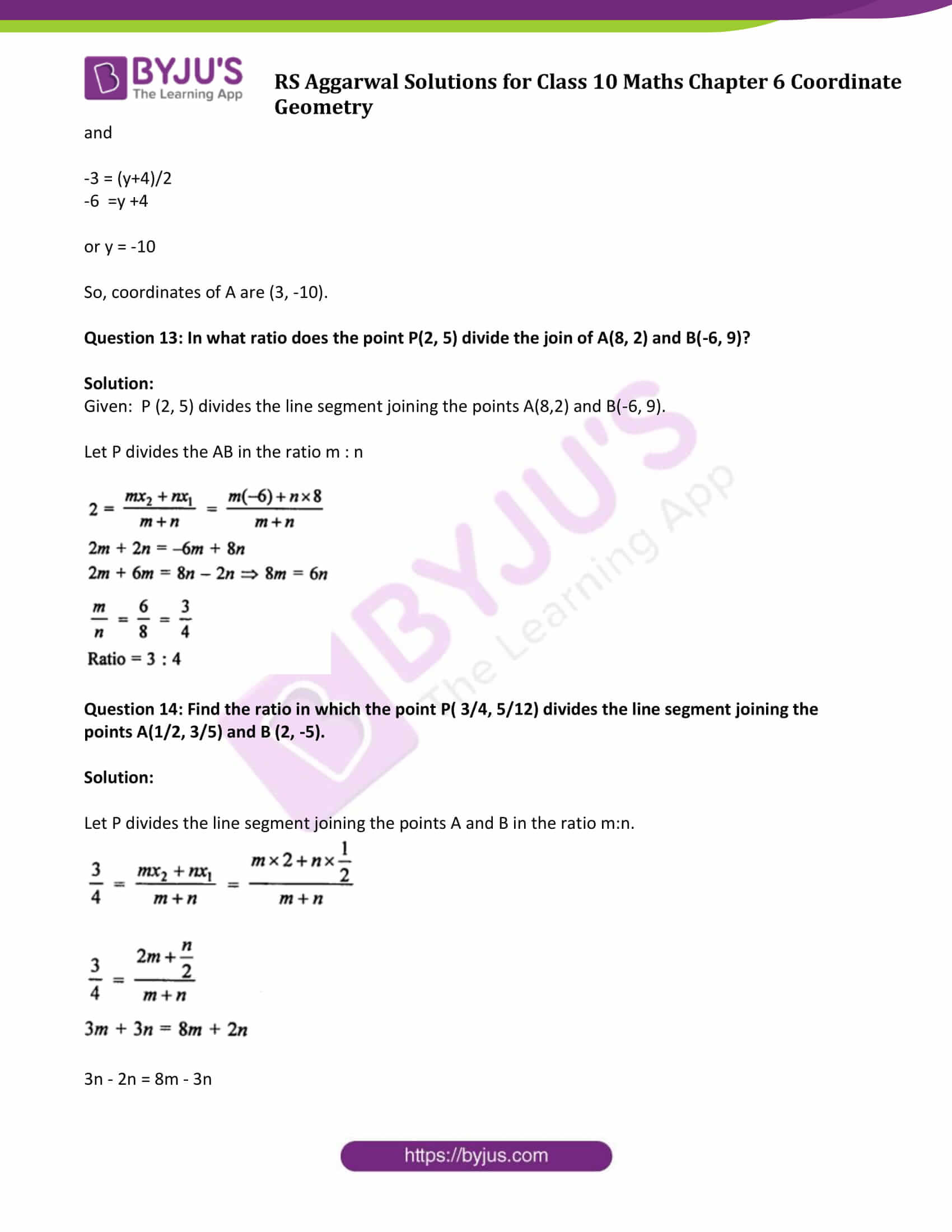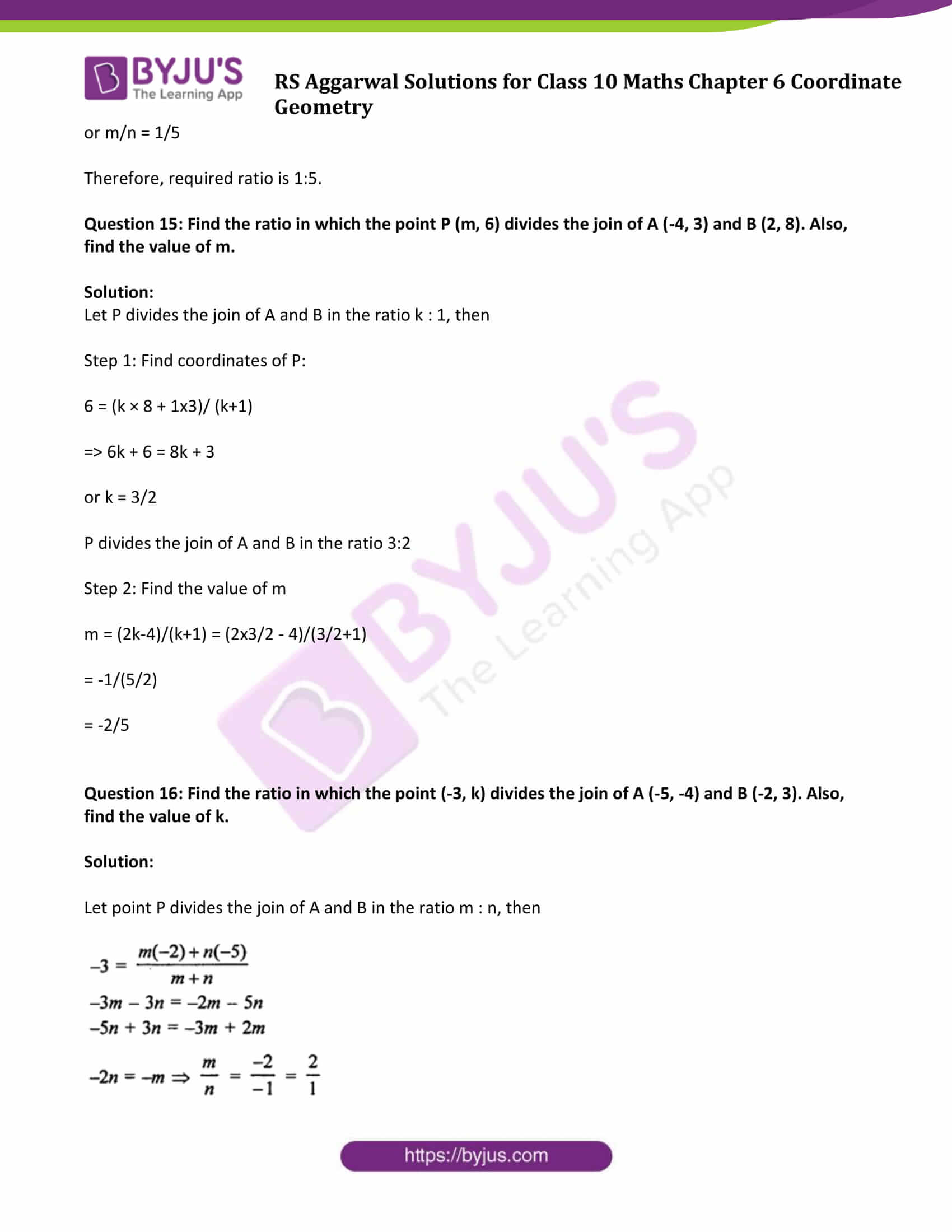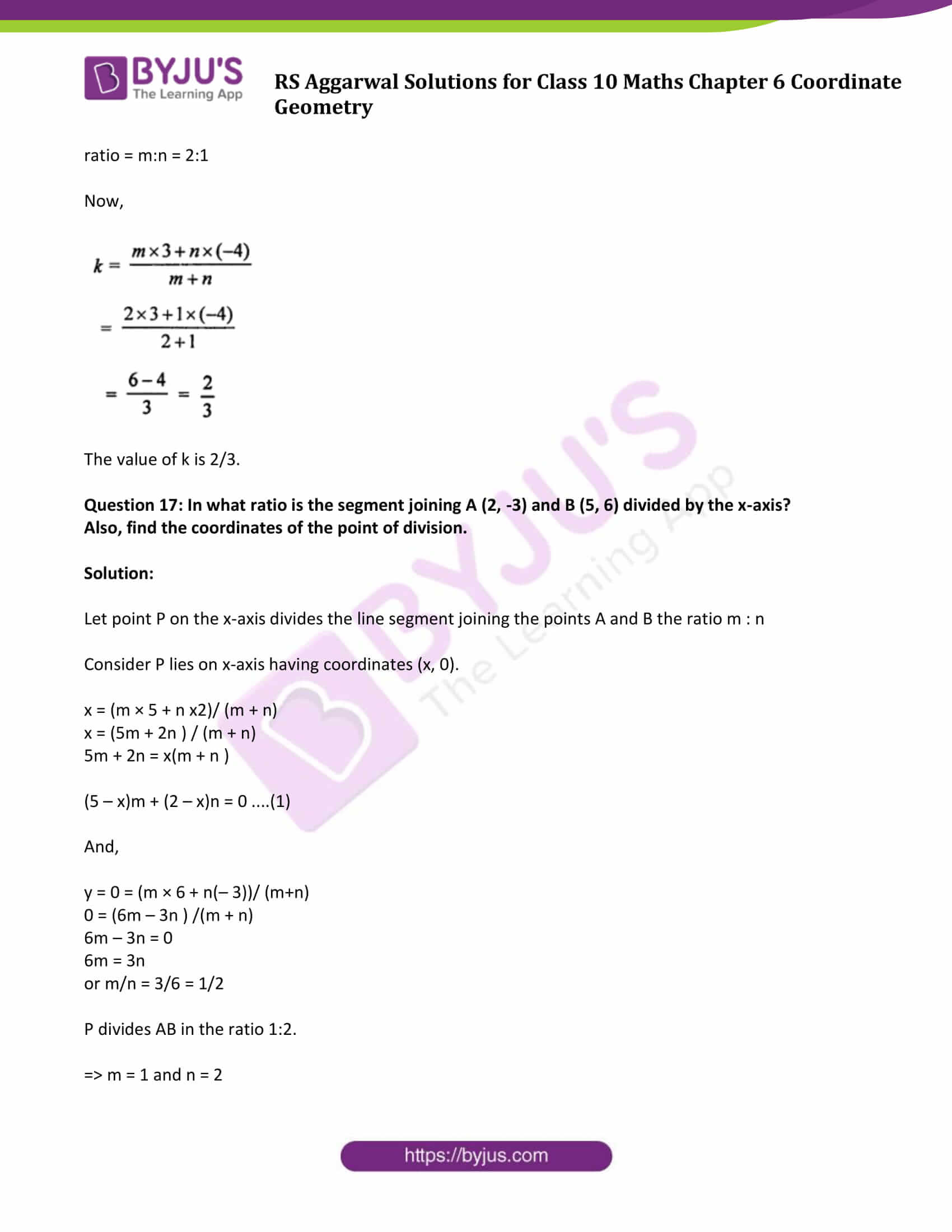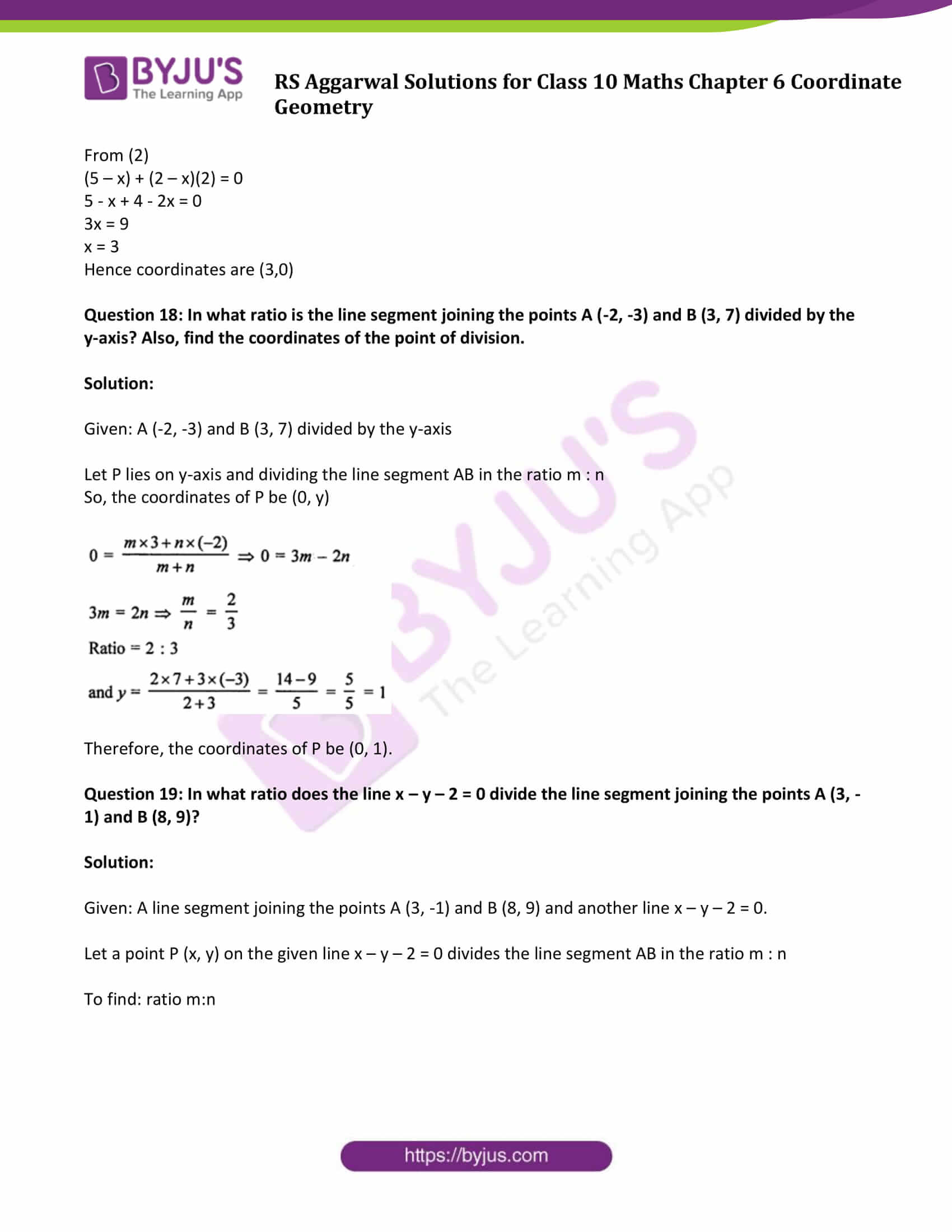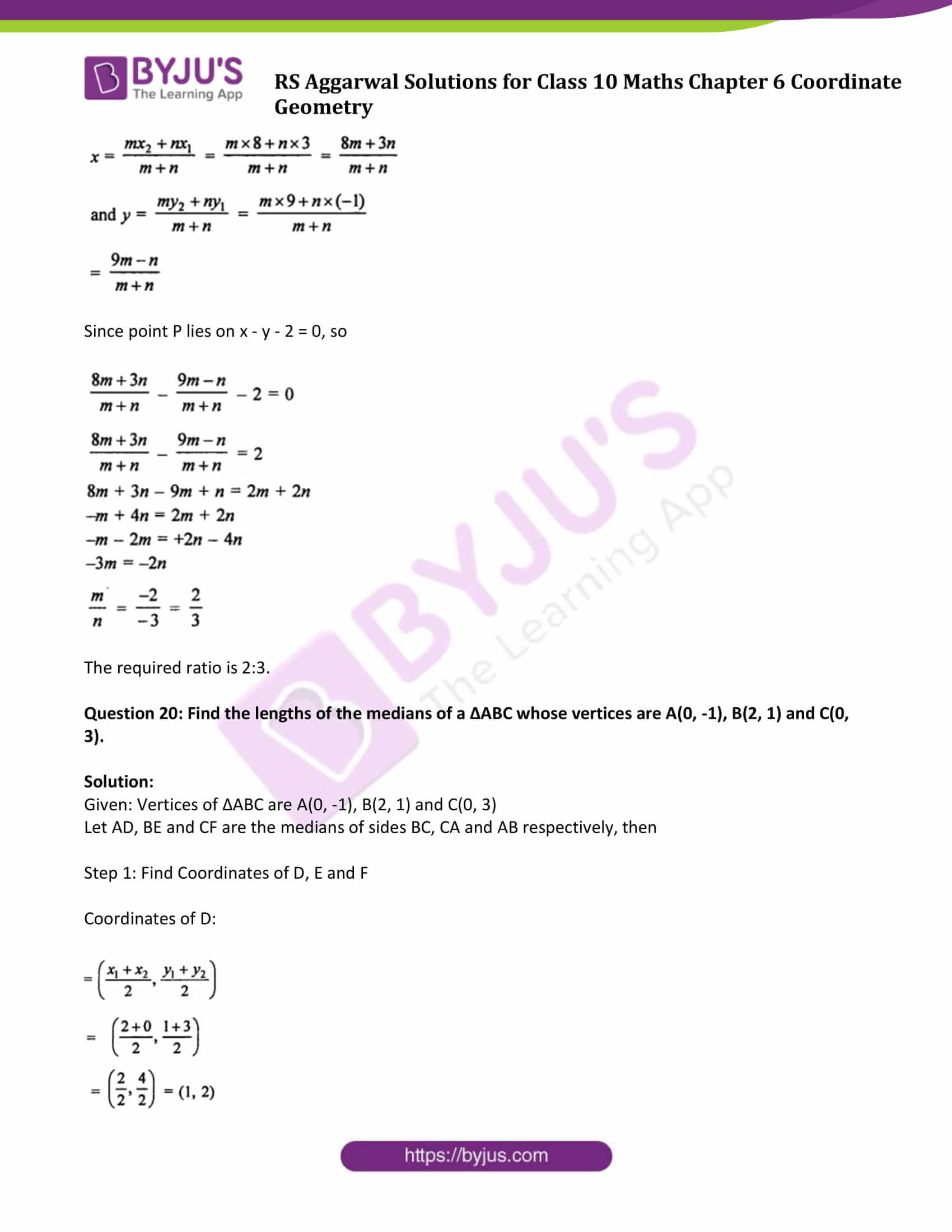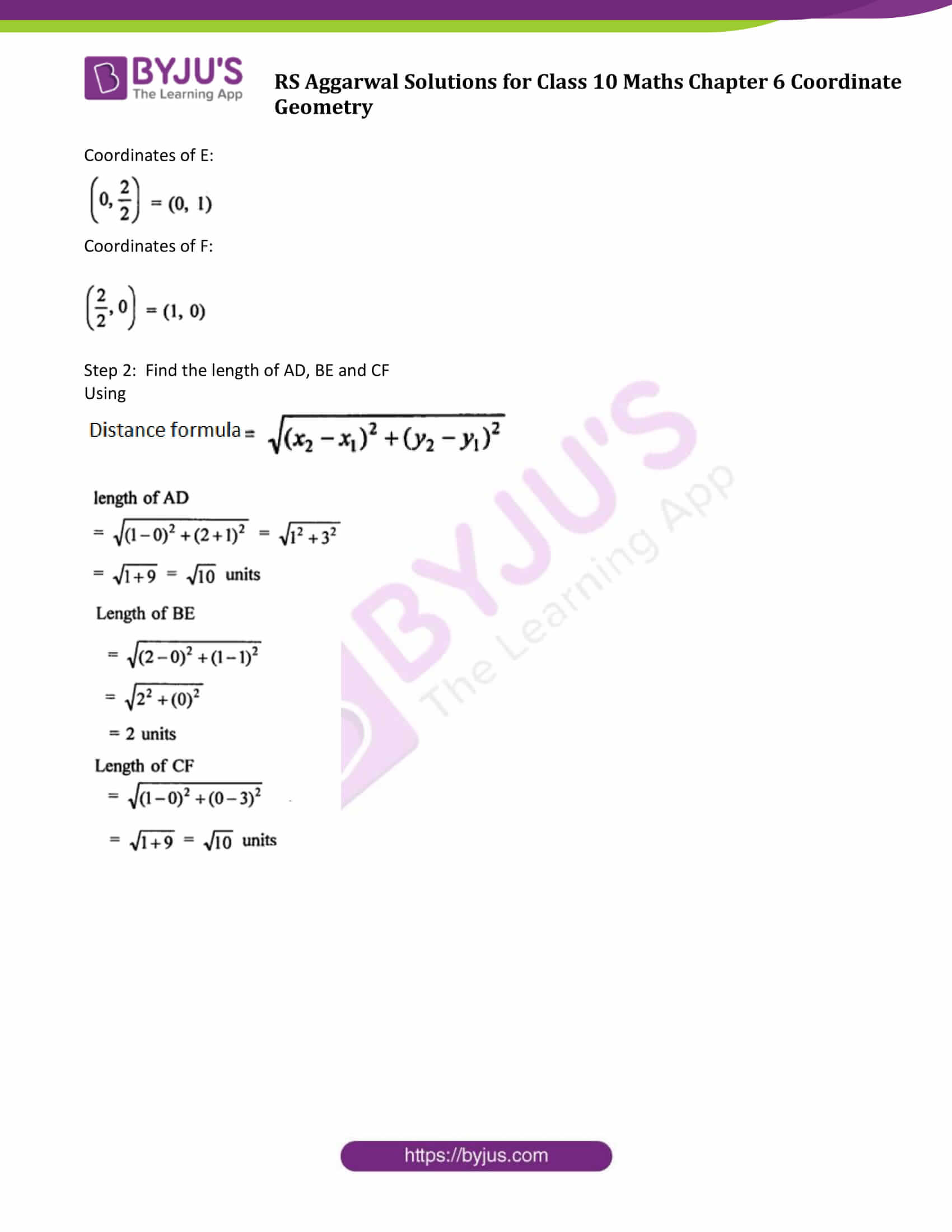### Access other exercise solutions of Class 10 Maths Chapter 6 Coordinate Geometry

Exercise 6A Solutions: 20 Questions (20 Short Answers)

Exercise 6C Solutions: 31 Questions (31 Long Answers)

Exercise 6D Solutions: 17 Questions (12 Short Answers & 5 Long Answers )

## Exercise 6B Page No: 320

Question 1:

(i) Find the coordinates of the point which divides the join of A (-1, 7) and B (4, -3) in the ratio 2 : 3.

(ii) Find the coordinates of the point which divides the join of A (-5, 11) and B (4, -7) in the ratio 7 : 2.

Solution:

If a point P(x,y) divides a line segment having end points coordinates (x1, y1) and (x2, y2), then coordinates of the point P can be find using below formula:

$x = \frac{m_{1}x_{2}+m_{2}x_{1}}{m_{1}+m_{2}}$ $y = \frac{m_{1}y_{2}+m_{2}y_{1}}{m_{1}+m_{2}}$

(i) Let P(x, y) be the point which divides the line joining the points A (-1, 7) and B (4, -3) in the ratio 2 : 3. then

x = (2 × 4 + 3 × (– 1))/(2 + 3)

= (8 – 3) /5

= 5/5

= 1

x = 1.

y = (2 × – 3 + 3 × 7)/ 5

= (– 6 + 21)/5

= 15 / 5

= 3

y = 3

Therefore, required point is (1, 3).

x = (7 × 4 + 2 × (– 5))/(7 + 2)

= (28 – 10) /9

= 18/9

= 2

y = (7 × (– 7) + 2 × 11)/ 9

= (– 49 + 22)/9

= – 27 / 9

= – 3

Therefore, required point is (2, – 3)

Question 2: Find the coordinates of the points of trisection of the line segment joining the points A (7, -2) and B (1, -5).

Solution:

Let A(7, -2) and B(1, -5) be the given points and P(x, y) and Q (x’, y’) are the points of trisection.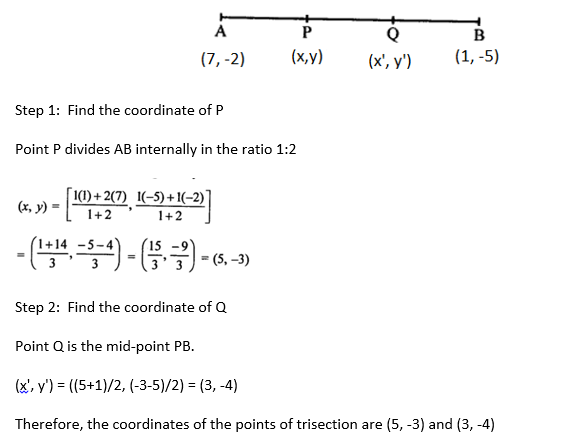Question 3: If the coordinates of points A and B are (-2, -2) respectively, find the coordinates of the point P such that AP = 3/7 AB, where P lies on the line segment AB.

Solution:

Coordinate of point P(x, y) can be calculated by using below formula:

Now,

$x = \frac{m_{1}x_{2}+m_{2}x_{1}}{m_{1}+m_{2}}$ $y = \frac{m_{1}y_{2}+m_{2}y_{1}}{m_{1}+m_{2}}$

x = ((3 × 2) + 4(– 2))/ (3 + 4)

= (6 – 8)/7

= – 2/7

y = (3(– 4) + 4(– 2))/ 7

= (– 12 – 8)/ 7

= – 20 / 7

Point P is (-2/7, -20/7)

Question 4: Point A lies on the line segment PQ joining P (6, -6) and Q (-4, -1) in such a way that PA/PQ = 2/5. If the point A also lies on the line 3x + k(y + 1) = 0, find the value of k.

Solution:

Let the point A(x, y) which lies on line joining P(6, -6) and Q(-4, -1) such that PA/PQ = 2/5

Line segment PQ is divided by the point A in the ratio 2:3.

$x = \frac{m_{1}x_{2}+m_{2}x_{1}}{m_{1}+m_{2}}$ $y = \frac{m_{1}y_{2}+m_{2}y_{1}}{m_{1}+m_{2}}$

Step 1: Find coordinates of A(x, y)

x = (2(– 4) + 3(6))/(2 + 3)

= (– 8 + 18) /5

= 10/5 = 2

y = (2(– 1) + 3(– 6))/ 5

= (–2 – 18)/5

= – 20 / 5

= – 4

Step 2: Point A also lies on the line 3x + k(y + 1) = 0

3(2) + k(– 4 + 1) = 0

6 – 3k = 0

or k = 2

Question 5: Points P, Q, R and S divide the line segment joining the points A (1, 2) and B (6, 7) in five equal parts. Find the coordinates of the points P, Q and R.

Solution:

Given: Points P, Q, R and S divides a line segment joining the points A (1, 2) and B (6, 7) in 5 equal parts.

We know that:

$x = \frac{m_{1}x_{2}+m_{2}x_{1}}{m_{1}+m_{2}}$ $y = \frac{m_{1}y_{2}+m_{2}y_{1}}{m_{1}+m_{2}}$

Now,

Step 1: Find coordinates of P.

P(x, y) divides AB in the ratio 1:4

x = (1 × 6 + 4 × 1)/1 + 4

= (6 + 4) /5

= 10/5

= 2

y = (1x 7 + 4 × 2)/5

= (7 + 8)/5

= 15 / 5

= 3

So, P(x, y) = P(2, 3)

Step 2: Find coordinates of Q.

Q divides the segment AB in ratio 2:3

x = (2x 6 + 3x 1)/5

= (12 + 3) /5

= 15/5 = 3

y = (2 × 7 + 3 × 2)/ 5

= (14 + 6)/5

= 20 / 5 = 4

So, Q(x, y) = Q(3,4)

Step 3: Find coordinates of R.

R divides the segment AB in ratio 3:2

x = (3 × 6 + 2 × 1)/5

= (18 + 2) /5

= 20/5

= 4

y = (3 × 7 + 2 × 2)/ 5

= (21 + 4)/5

= 25 / 5

= 5

So, R(x, y) = R(4,5)

Question 6: Points P, Q and R in that order are dividing a line segment joining A (1, 6) and B (5, -2) in four equal parts. Find the coordinates of P, Q and R.

Solution:

Given: Points P, Q and R in order divide a line segment joining the points A (1, 6) and B (5, -2) in four equal parts.

Using formulas:

$x = \frac{m_{1}x_{2}+m_{2}x_{1}}{m_{1}+m_{2}}$ $y = \frac{m_{1}y_{2}+m_{2}y_{1}}{m_{1}+m_{2}}$

Step 1: Find coordinates of P.

P(x, y) divides AB in the ratio of 1:3

x = (5+3)/4 = 8/4 = 2

y = (-2+18) = 16/4 = 4

So P(x, y) = P(2, 4)

Step 2: Find coordinates of Q.

Q divides the segment AB in ratio 2:2 or 1:1. So Q ia midpoint of AB

So Q((1+5)/2, (6-2)/2) = (3, 2)

So, Q(x, y) = Q(3,2)

Step 3: Find coordinates of R.

R divides the segment AB in ratio 3:1

x = (3 × 5 + 1 × 1)/4

= 4

y = (3x(-2) + 1×6)/4

= 0

So, R(x, y) = R(4,0)

Question 7: The line segment joining the points A (3, -4) and B (1, 2) is trisected at the points P(p, -2) and Q(1/2, q). Find the values of p and q.

Solution:

The line segment joining the point A(3, -4) and B(1, 2) is trisected by the points P(p, -2) and Q( 1/2, q). (given)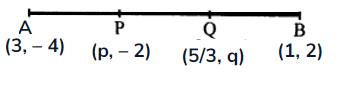Step 1: Find x coordinate of P which is p

P(p, -2) divides AB in the ratio of 1:2

p = (1+6)/3 = 7/3

Step 2: Find coordinates of Q

Q divides the segment AB in ratio 2:1

x = (2×1 + 1×3)/3

= (2 + 3) /3

= 5/3

y = (2×2 + 1(– 4))/3

= (4 – 4)/3

= 0/ 3

= 0

= q

Therefore, p = 7/3 and q = 0

Question 8: Find the coordinates of the midpoint of the line segment joining

(i) A (3, 0) and B (-5, 4)

(ii) P (-11, -8) and Q (8, -2)

Solution:

(i) Midpoint of the line segment joining A (3, 0) and B (-5, 4):

Midpoint = ((x_1 + x_2)/2 , (y_1+y_2)/2)

= ((3-5)/2, (0+4)/2)

= (-1, 2)

(ii) Midpoint of the line segment joining P (-11, -8) and Q (8, -2)

PQ midpoint = ((-11+8)/2, (-8-2)/2)

= (-3/2, -5)

Question 9: If (2, p) is the midpoint of the line segment joining the points A (6, -5) and B (-2, 11), find the value of p.

Solution:

Given: (2, p) is the mid point of the line segment joining the points A (6, -5), B (-2, 11)

To find: the value of p

p = (-5+11)/2 = 6/2 = 3

Question 10: The midpoint of the line segment joining A (2a, 4) and B (-2, 3b) is C (1, 2a + 1). Find the values of a and b.

Solution:

Mid point of the line segment joining the points A(2a, 4) and B (-2, 3b) is C(1, 2a + 1)

Mid point of AB = ( (2a-2)/2, (4+3b)/2 ) ….(1)

Mid point of AB = (1, 2a + 1) ….(2) (given)

Now, from (1) and (2)

1 = (2a-2)/2

=> a = 2

and 2a + 1 = (4+3b)/2

10-4 = 3b

or b = 2

Answer: a = 2 and b = 2

Question 11: The line segment joining A(-2, 9) and B(6, 3) is a diameter of a circle with centre C. Find the coordinates of C.

Solution:

The line segment joining the points A(-2, 9) and B(6, 3) is a diameter of a circle with centre C.

Which means C is the midpoint of AB.

let (x, y) be the coordinates of C, then

x = (-2 + 6)/2 = 2 and

y = (9+3)/2 = 6

So, coordinates of C are (2, 6).

Question 12: Find the coordinates of a point A, where AB is a diameter of a circle with centre C(2, -3) and the other end of the diameter is B (1, 4).

Solution:

Given:

AB is diameter of a circle with centre C.

Coordinates of C(2, -3) and other point is B (1, 4)

Point C is the midpoint of AB.

Let (x, y) be the coordinates of A, then

2 = (x+1)/2

4 = x +1

x = 3

and

-3 = (y+4)/2

-6 =y +4

or y = -10

So, coordinates of A are (3, -10).

Question 13: In what ratio does the point P(2, 5) divide the join of A(8, 2) and B(-6, 9)?

Solution:

Given: P (2, 5) divides the line segment joining the points A(8,2) and B(-6, 9).

Let P divides the AB in the ratio m : n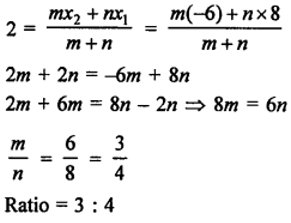Question 14: Find the ratio in which the point P( 3/4, 5/12) divides the line segment joining the points A(1/2, 3/5) and B (2, -5).

Solution:

Let P divides the line segment joining the points A and B in the ratio m:n.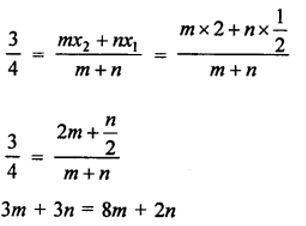3n – 2n = 8m – 3n

or m/n = 1/5

Therefore, required ratio is 1:5.

Question 15: Find the ratio in which the point P (m, 6) divides the join of A (-4, 3) and B (2, 8). Also, find the value of m.

Solution:

Let P divides the join of A and B in the ratio k : 1, then

Step 1: Find coordinates of P:

6 = (k × 8 + 1×3)/ (k+1)

=> 6k + 6 = 8k + 3

or k = 3/2

P divides the join of A and B in the ratio 3:2

Step 2: Find the value of m

m = (2k-4)/(k+1) = (2×3/2 – 4)/(3/2+1)

= -1/(5/2)

= -2/5

Question 16: Find the ratio in which the point (-3, k) divides the join of A (-5, -4) and B (-2, 3). Also, find the value of k.

Solution:

Let point P divides the join of A and B in the ratio m : n, then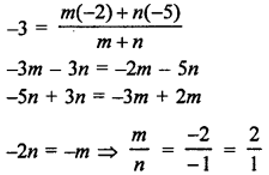ratio = m:n = 2:1

Now,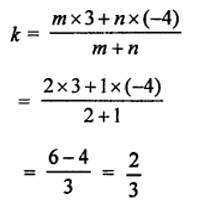The value of k is 2/3.

Question 17: In what ratio is the segment joining A (2, -3) and B (5, 6) divided by the x-axis?

Also, find the coordinates of the point of division.

Solution:

Let point P on the x-axis divides the line segment joining the points A and B the ratio m : n

Consider P lies on x-axis having coordinates (x, 0).

x = (m × 5 + n x2)/ (m + n)

x = (5m + 2n ) / (m + n)

5m + 2n = x(m + n )

(5 – x)m + (2 – x)n = 0 ….(1)

And,

y = 0 = (m × 6 + n(– 3))/ (m+n)

0 = (6m – 3n ) /(m + n)

6m – 3n = 0

6m = 3n

or m/n = 3/6 = 1/2

P divides AB in the ratio 1:2.

=> m = 1 and n = 2

From (2)

(5 – x) + (2 – x)(2) = 0

5 – x + 4 – 2x = 0

3x = 9

x = 3

Hence coordinates are (3,0)

Question 18: In what ratio is the line segment joining the points A (-2, -3) and B (3, 7) divided by the y-axis? Also, find the coordinates of the point of division.

Solution:

Given: A (-2, -3) and B (3, 7) divided by the y-axis

Let P lies on y-axis and dividing the line segment AB in the ratio m : n

So, the coordinates of P be (0, y)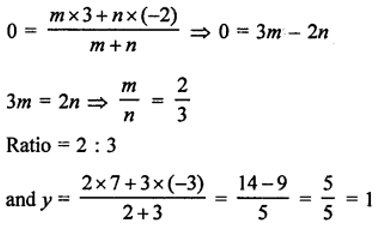Therefore, the coordinates of P be (0, 1).

Question 19: In what ratio does the line x – y – 2 = 0 divide the line segment joining the points A (3, -1) and B (8, 9)?

Solution:

Given: A line segment joining the points A (3, -1) and B (8, 9) and another line x – y – 2 = 0.

Let a point P (x, y) on the given line x – y – 2 = 0 divides the line segment AB in the ratio m : n

To find: ratio m:n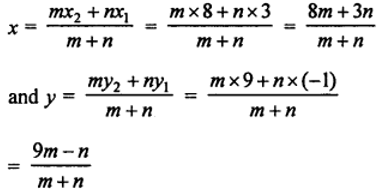Since point P lies on x – y – 2 = 0, so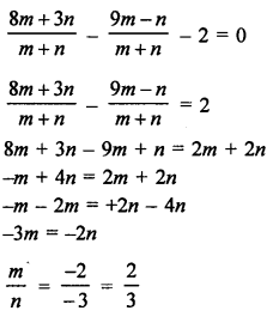The required ratio is 2:3.

Question 20: Find the lengths of the medians of a ∆ABC whose vertices are A(0, -1), B(2, 1) and C(0, 3).

Solution:

Given: Vertices of ∆ABC are A(0, -1), B(2, 1) and C(0, 3)

Let AD, BE and CF are the medians of sides BC, CA and AB respectively, then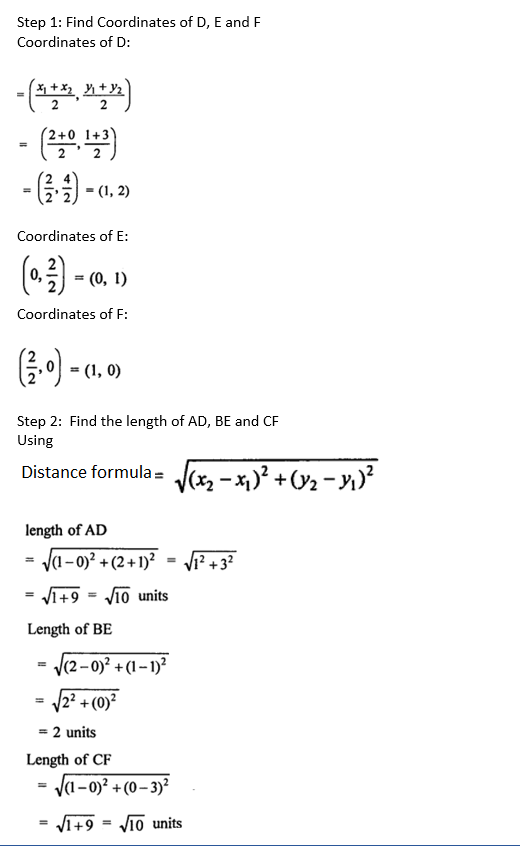## R S Aggarwal Solutions for Class 10 Maths Chapter 6 Coordinate Geometry Exercise 6B Topics

Class 10 Maths Chapter 6 Coordinate Geometry Exercise 6B is based on following topics:

• Section formula.

The coordinates of the point A(x, y) which divides the line segment joining P(x1, y1) and Q(x2, y2) internally in the ratio m:n are given by: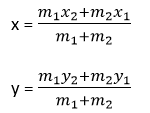• Midpoint Formula

The coordinates of the midpoint M of a line segment PQ with endpoints P(x1, y1) and Q(x2, y2) are

Midpoint = ((x1 + x2)/2 , (y1+y2)/2)

• Centroid of a triangle

The point of intersection of the medians of a triangle is called as centroid.